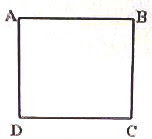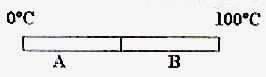## Pages

`“Life is like riding a bicycle.  To keep your balance you must keep moving.”–Albert Einstein`

## Tuesday, February 10, 2009

### AP Physics B Multiple Choice Practice Questions on Temperature and Heat

The pursuit of truth and beauty is a sphere of activity in which we are permitted to remain children all our lives

– Albert Einstein

As promised in the last post dated 6th February 2009, I give below a few multiple choice practice questions on temperature and heat relevant to AP Physics B:

(1) A soft iron rod has its length increased by 0.3% on increasing its temperature by ∆T. The percentage increase in the area of a circular hole in a sheet of the same material (soft iron) on increasing its temperature by ∆T will be

(a) 0.3%

(b) 0.3%

(c) 0%

(d) 0.6%

(e) –0.6%

The radius of the hole will increase by 0.3% and hence the area of the hole will increase by 0.6%.

[The area A = πR2 where R is the radius. Therefore, ∆A/A =2 ∆R/R. The fractional change in area is equal to twice the fractional change in radius. Therefore, the percentage change in area is equal to twice the percentage change in radius].

(2) The temperature of oil in a vessel is measured using a Celsius scale thermometer as well as a Fahrenheit scale thermometer. The readings are respectively tº C and 2tº F. The temperature of the oil is

(a) 32º C

(b) 100º C

(c) 120º C

(d) 142º C

(e) 160º C

We have tC/100 = (tF – 32) /180.

[This can be remembered as tC = (5/9)(tF – 32)]

Here tC = t and tF = 2t so that t/100 = (2t– 32) /180.

Or, 9t = 10t– 160 from which t = 160.

Therefore, the temperature of oil is 160º C.

(3) A copper rod and a steel rod are to have lengths Lc and Ls such that the difference between their lengths is the same at all ambient temperatures. If the coefficients of linear expansion of copper and steel are respectively αc and αs the lengths are related to the coefficients of linear expansion as

(a) Lc/Ls = αc/αs

(b) Lc Ls = αc αs

(c) Lc/Ls = αs/αc

(d) Lc/Ls = (αc/αs)1/2

(e) Lc/Ls = (αc/αs)–1/2

The changes in length must be equal on heating (or cooling) so that we have

Lc αc ∆T = Ls αs ∆T

This gives Lc/Ls = αs/αc(4) Four identical brass rods AB, BC, CD and DA are joined to form a square (fig). If the diagonally opposite corners A and C are kept in melting ice and boiling water respectively what is the temperature difference between the corners B and D?

(a) 50º C

(b) 100º C

(c) 25º C

(d) 75º C

(e) 0º C

The corners B and D will be at the same temperature so that the difference will be zero.(5) Thermal conductivity of metal A is three times the thermal conductivity of metal B. Two rods of the same dimensions made of metals A and B are joined end to end (fig.). The free end of A is maintained at 0º C and that of of B is maintained at 100º C. The temperature of the junction between A and B in the steady state will be

(a) 25º C

(b) 33.3º C

(c) 66.6º C

(d) 75º C

(e) 90º C

In the steady state the quantity of heat flowing through B per second will be equal to that flowing through A per second. If the temperature of the junction between A and B is tº C we have

kA(100 – t)/L = 3kA(t – 0)/L where A is the area of cross section, L is the length, k is the thermal conductivity of B and therefore 3k is the thermal conductivity of A.

Therefore 100 – t = 3t so that t = 25º C

[Since the rods are of identical dimensions and the thermal conductivity of A is three times that of B, you can easily argue that the temperature drop in A is only one-third of the temperature drop in B and arrive at the answer].

(6) A cup of coffee cools from 65º C to 63º C in one minute in a room of temperature 24º C. To cool from 58º C to 50º C the same cup of coffee will take nearly

(a) 2 min.

(b) 2.4 min.

(c) 3.8 min.

(d) 4.5 min

(e) 5.3 min

Since the rate of cooling is directly proportional to the excess of temperature (in accordance with Newton’s law of cooling), we have

S(65 – 63)/1 α (64 – 24) and

S(58 – 50)/t α (54 – 24)

where S is the heat capacity (thermal capacity) of the cup of coffee (which is the heat required to raise the temperature through 1K) and t is the time required to cool from 58º C to 50º C. Note that we have used the average temperatures (64º C and 54º C) of the cup of coffee to find the mean excess temperatures in the two cases.

On dividing, 2t/8 = 40/30 from which t = 5.3 min (nearly)